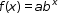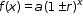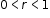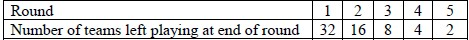# MA.912.AR.5.6Export Print
Given a table, equation or written description of an exponential function, graph that function and determine its key features.

### Clarifications

Clarification 1: Key features are limited to domain; range; intercepts; intervals where the function is increasing, decreasing, positive or negative; constant percent rate of change; end behavior and asymptotes.

Clarification 2: Instruction includes representing the domain and range with inequality notation, interval notation or set-builder notation.

Clarification 3: Within the Algebra 1 course, notations for domain and range are limited to inequality and set-builder.

Clarification 4: Within the Algebra 1 course, exponential functions are limited to the forms, where b is a whole number greater than 1 or a unit fraction or, where.

General Information
Subject Area: Mathematics (B.E.S.T.)
Strand: Algebraic Reasoning
Status: State Board Approved

## Benchmark Instructional Guide

### Terms from the K-12 Glossary

• Coordinate plane
• Domain
• Exponential Function
• Function Notation
• Range
• $x$-intercept
• $y$-intercept

### Vertical Alignment

Previous Benchmarks

Next Benchmarks

### Purpose and Instructional Strategies

In grade 8, students graphed linear equations. In Algebra I, students graph exponential functions and determine their key features, including asymptotes and end behavior. Students are first introduced to asymptotes in Algebra I. In later courses, asymptotes are important in the study of other types of functions, including rational functions.
• Instruction provides the opportunity for students to explore the meaning of an asymptote graphically and algebraically. Through work in this benchmark, students will discover asymptotes are useful guides to complete the graph of a function, especially when drawing them by hand. For mastery of this benchmark, asymptotes can be drawn on the graph as a dotted line or not drawn on the graph.
• For students to have full understanding of exponential functions, instruction includes MA.912.AR.5.3 and MA.912.AR.5.4. Growth or decay of a function can be defined as a key feature (constant percent rate of change) of an exponential function and useful in understanding the relationships between two.
• Instruction includes the use of $x$-$y$ notation and function notation.
• Instruction includes representing domain and range using words, inequality notation and set-builder notation.
• Words If the domain is all real numbers, it can be written as “all real numbers” or “any value of $x$, such that $x$ is a real number.”
• Inequality notation If the domain is all values of $x$ greater than 2, it can be represented as $x$ > 2.
• Set-builder notation If the domain is all values of $x$ less than or equal to zero, it can be represented as {$x$|$x$ ≤ 0} and is read as “all values of $x$ such that $x$ is less than or equal to zero.”
• Instruction includes the use of appropriately scaled coordinate planes, including the use of breaks in the $x$- or $y$-axis when necessary.

### Common Misconceptions or Errors

• Students may not fully understand how to use proper notation when determining the key features of an exponential function.

### Strategies to Support Tiered Instruction

• Instruction includes student understanding that growth and decay is not the same as a function increasing or decreasing.
• For example, the exponential function $y$ = −2(0.5)$x$ is an exponential decay function because the value of $b$ is in between 0 and 1. Note that it is the magnitudes of the $y$-values that are decaying exponentially, eventually getting closer to zero. However, the value of the function increases as the value of $x$ increases. To help students visualize this, graph the function using graphing technology.
• Instruction includes using an exponential function formula guide like the one below.
Exponential Growth Exponential Decay
$b$ > 1                 $b$ < 1
$y$ = $a$(1 + $r$)$t$ $y$ = $a$(1 − $r$)$t$

• The bracket system for the NCAA Basketball Tournament (also known as March Madness) is an example of an exponential function. At each round of the tournament, teams play against one another with only the winning teams progressing to the next round. If we start with 64 teams going into round 1, the table of values looks something like this:• Part A. Graph this function.
• Part B. What is the percentage of teams left after each round?

• Ashanti purchased a car for \$22,900. The car depreciated at an annual rate of 16%. After 5 years, Ashanti wants to sell her car.
• Part A. Write an equation that models the value of Ashanti’s car?
• Part B. What would be the range of the graph of the value of Ashanti’s car?
• Part C. What would be the $y$-intercept of that graph and what does it represent?
• Part D. Will her car ever have a value of \$0.00 based on your equation?
• Part E. What would be a sensible domain for this function? Justify your answer.

### Instructional Items

Instructional Item 1
• An exponential function is given by the equation $y$ = −14($\frac{\text{1}}{\text{4}}$)$x$. What is the asymptote for 4 the graph?

Instructional Item 2
• An exponential function is given.
$y$ = 50(1.1)$t$
• Part A. Does this function represent exponential growth or decay?
• Part B. What is the constant percent rate of change of $y$ with respect to $t$.

*The strategies, tasks and items included in the B1G-M are examples and should not be considered comprehensive.

## Related Courses

This benchmark is part of these courses.
1200310: Algebra 1 (Specifically in versions: 2014 - 2015, 2015 - 2022, 2022 and beyond (current))
1200320: Algebra 1 Honors (Specifically in versions: 2014 - 2015, 2015 - 2022, 2022 and beyond (current))
1200380: Algebra 1-B (Specifically in versions: 2014 - 2015, 2015 - 2022, 2022 and beyond (current))
1200400: Foundational Skills in Mathematics 9-12 (Specifically in versions: 2014 - 2015, 2015 - 2022, 2022 and beyond (current))
7912070: Access Mathematics for Liberal Arts (Specifically in versions: 2014 - 2015, 2015 - 2018, 2018 - 2019, 2019 - 2022, 2022 - 2023, 2023 and beyond (current))
7912090: Access Algebra 1B (Specifically in versions: 2014 - 2015, 2015 - 2018, 2018 - 2019, 2019 - 2022, 2022 and beyond (current))
1200315: Algebra 1 for Credit Recovery (Specifically in versions: 2014 - 2015, 2015 - 2022, 2022 and beyond (current))
1200385: Algebra 1-B for Credit Recovery (Specifically in versions: 2014 - 2015, 2015 - 2022, 2022 and beyond (current))
7912075: Access Algebra 1 (Specifically in versions: 2014 - 2015, 2015 - 2018, 2018 - 2019, 2019 - 2022, 2022 and beyond (current))
1207350: Mathematics for College Liberal Arts (Specifically in versions: 2022 and beyond (current))
1200710: Mathematics for College Algebra (Specifically in versions: 2022 and beyond (current))

## Related Access Points

Alternate version of this benchmark for students with significant cognitive disabilities.
MA.912.AR.5.AP.6: Given a table, equation or written description of an exponential function, select the graph that represents the function.

## Related Resources

Vetted resources educators can use to teach the concepts and skills in this benchmark.

## Formative Assessments

Graphing an Exponential Function:

Students are asked to graph an exponential function and to determine if the function is an example of exponential growth or decay, describe any intercepts, and describe the end behavior of the graph.

Type: Formative Assessment

Comparing Functions - Exponential:

Students are asked to use technology to graph exponential functions and then to describe the effect on the graph of changing the parameters of the function.

Type: Formative Assessment

Loss of Fir Trees:

Students are asked to sketch a graph that depicts the exponential decline in the population of fir trees in a forest.

Type: Formative Assessment

## Lesson Plan

Which Function?:

This activity has students apply their knowledge to distinguish between numerical data that can be modeled in linear or exponential forms. Students will create mathematical models (graph, equation) that represent the data and compare these models in terms of the information they show and their limitations. Students will use the models to compute additional information to predict future outcomes and make conjectures based on these predictions.

Type: Lesson Plan

## Original Student Tutorials

Exponential Functions Part 3: Decay:

Learn about exponential decay as you calculate the value of used cars by examining equations, graphs, and tables in this interactive tutorial.

Type: Original Student Tutorial

Exponential Functions Part 2: Growth:

Learn about exponential growth in the context of interest earned as money is put in a savings account by examining equations, graphs, and tables in this interactive tutorial.

Type: Original Student Tutorial

Exponential Functions Part 1:

Learn about exponential functions and how they are different from linear functions by examining real world situations, their graphs and their tables in this interactive tutorial.

Type: Original Student Tutorial

## Tutorial

Graphing Exponential Equations:

This tutorial will help you to learn about exponential functions by graphing various equations representing exponential growth and decay.

Type: Tutorial

## MFAS Formative Assessments

Comparing Functions - Exponential:

Students are asked to use technology to graph exponential functions and then to describe the effect on the graph of changing the parameters of the function.

Graphing an Exponential Function:

Students are asked to graph an exponential function and to determine if the function is an example of exponential growth or decay, describe any intercepts, and describe the end behavior of the graph.

Loss of Fir Trees:

Students are asked to sketch a graph that depicts the exponential decline in the population of fir trees in a forest.

## Original Student Tutorials Mathematics - Grades 9-12

Exponential Functions Part 1:

Learn about exponential functions and how they are different from linear functions by examining real world situations, their graphs and their tables in this interactive tutorial.

Exponential Functions Part 2: Growth:

Learn about exponential growth in the context of interest earned as money is put in a savings account by examining equations, graphs, and tables in this interactive tutorial.

Exponential Functions Part 3: Decay:

Learn about exponential decay as you calculate the value of used cars by examining equations, graphs, and tables in this interactive tutorial.

## Student Resources

Vetted resources students can use to learn the concepts and skills in this benchmark.

## Original Student Tutorials

Exponential Functions Part 3: Decay:

Learn about exponential decay as you calculate the value of used cars by examining equations, graphs, and tables in this interactive tutorial.

Type: Original Student Tutorial

Exponential Functions Part 2: Growth:

Learn about exponential growth in the context of interest earned as money is put in a savings account by examining equations, graphs, and tables in this interactive tutorial.

Type: Original Student Tutorial

Exponential Functions Part 1:

Learn about exponential functions and how they are different from linear functions by examining real world situations, their graphs and their tables in this interactive tutorial.

Type: Original Student Tutorial

## Tutorial

Graphing Exponential Equations:

This tutorial will help you to learn about exponential functions by graphing various equations representing exponential growth and decay.

Type: Tutorial

## Parent Resources

Vetted resources caregivers can use to help students learn the concepts and skills in this benchmark.